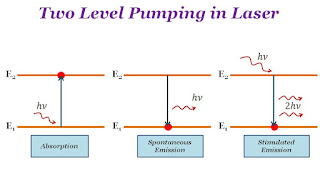### Two Level Pumping in Laser

Two-level pumping occurs between two energy levels. All the process of laser (absorption, spontaneous emission, or stimulated emission) occurs between two energy level. The absorption of light or emission of light energy is the difference between two energy levels. If two energy levels are $E_{1}$ and $E_{2}$ so absorption or emission of a photon →

 $E_{2}-E_{1}=h\nu$

Where$h$ → Planck's Constant$\nu$ → Frequency of photon

Two-level pumping in laser is not suitable for attaining the population inversion. The transition of atoms between two energy levels by stimulated emission is called a lasing transition. The lower level is known as the lower lasing level and the upper level is known as the upper lasing level. The upper lasing level must be a metastable level. The uppermost level to which atoms are in the excited state is known as the pumping level. The transition between the ground level and pumping level is called the pumping transition.Two-level pumping Laser